Research papers and code for "Carola Doerr":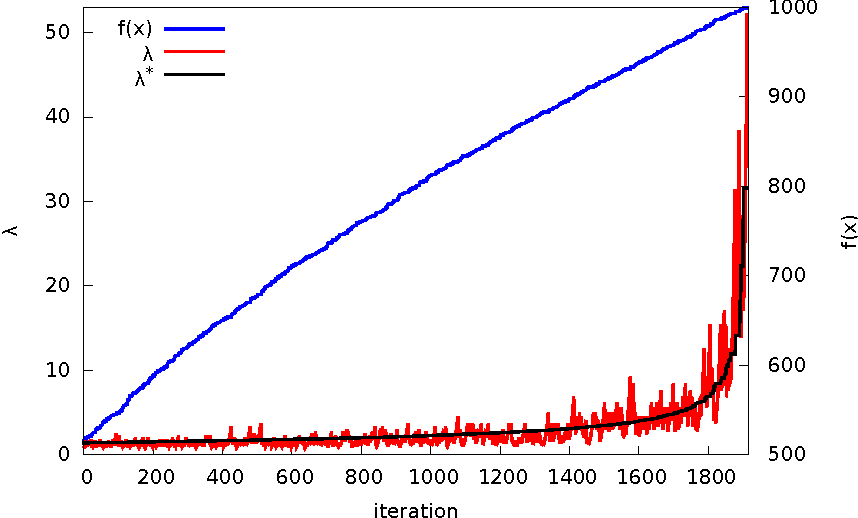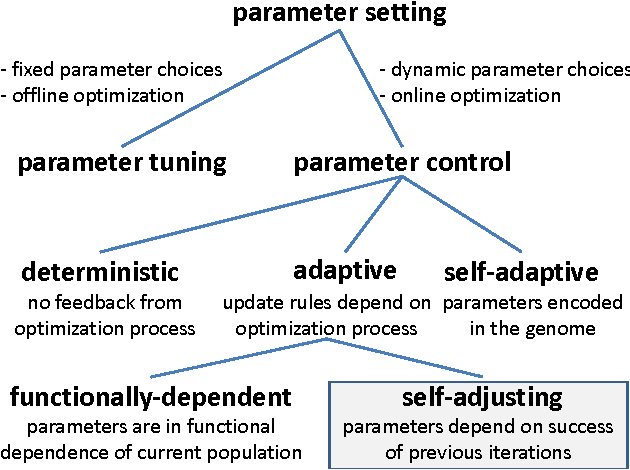While evolutionary algorithms are known to be very successful for a broad range of applications, the algorithm designer is often left with many algorithmic choices, for example, the size of the population, the mutation rates, and the crossover rates of the algorithm. These parameters are known to have a crucial influence on the optimization time, and thus need to be chosen carefully, a task that often requires substantial efforts. Moreover, the optimal parameters can change during the optimization process. It is therefore of great interest to design mechanisms that dynamically choose best-possible parameters. An example for such an update mechanism is the one-fifth success rule for step-size adaption in evolutionary strategies. While in continuous domains this principle is well understood also from a mathematical point of view, no comparable theory is available for problems in discrete domains. In this work we show that the one-fifth success rule can be effective also in discrete settings. We regard the $(1+(\lambda,\lambda))$~GA proposed in [Doerr/Doerr/Ebel: From black-box complexity to designing new genetic algorithms, TCS 2015]. We prove that if its population size is chosen according to the one-fifth success rule then the expected optimization time on \textsc{OneMax} is linear. This is better than what \emph{any} static population size $\lambda$ can achieve and is asymptotically optimal also among all adaptive parameter choices.

* This is the full version of a paper that is to appear at GECCO 2015
Click to Read Paper and Get Code
Understanding how crossover works is still one of the big challenges in evolutionary computation research, and making our understanding precise and proven by mathematical means might be an even bigger one. As one of few examples where crossover provably is useful, the $(1+(\lambda, \lambda))$ Genetic Algorithm (GA) was proposed recently in [Doerr, Doerr, Ebel: TCS 2015]. Using the fitness level method, the expected optimization time on general OneMax functions was analyzed and a $O(\max\{n\log(n)/\lambda, \lambda n\})$ bound was proven for any offspring population size $\lambda \in [1..n]$. We improve this work in several ways, leading to sharper bounds and a better understanding of how the use of crossover speeds up the runtime in this algorithm. We first improve the upper bound on the runtime to $O(\max\{n\log(n)/\lambda, n\lambda \log\log(\lambda)/\log(\lambda)\})$. This improvement is made possible from observing that in the parallel generation of $\lambda$ offspring via crossover (but not mutation), the best of these often is better than the expected value, and hence several fitness levels can be gained in one iteration. We then present the first lower bound for this problem. It matches our upper bound for all values of $\lambda$. This allows to determine the asymptotically optimal value for the population size. It is $\lambda = \Theta(\sqrt{\log(n)\log\log(n)/\log\log\log(n)})$, which gives an optimization time of $\Theta(n \sqrt{\log(n)\log\log\log(n)/\log\log(n)})$. Hence the improved runtime analysis gives a better runtime guarantee along with a better suggestion for the parameter $\lambda$. We finally give a tail bound for the upper tail of the runtime distribution, which shows that the actual runtime exceeds our runtime guarantee by a factor of $(1+\delta)$ with probability $O((n/\lambda^2)^{-\delta})$ only.

* This is a preliminary version of a paper that is to appear at Genetic and Evolutionary Computation Conference (GECCO 2015)
Click to Read Paper and Get Code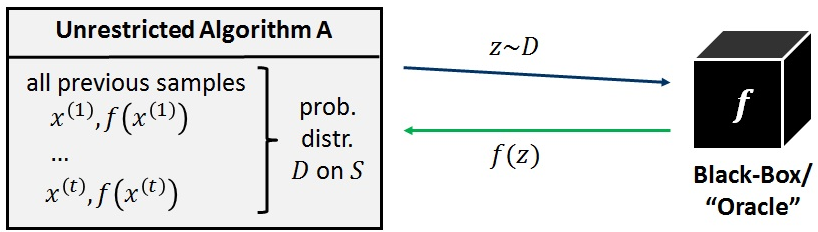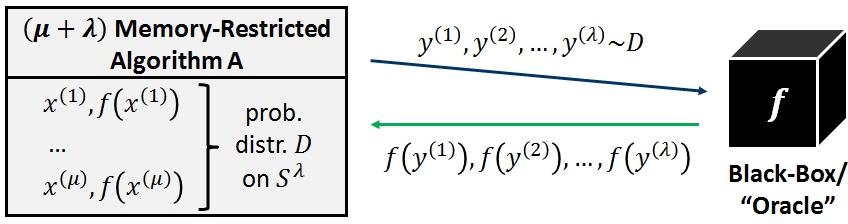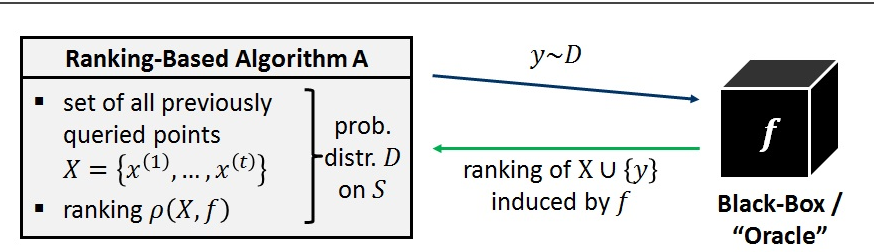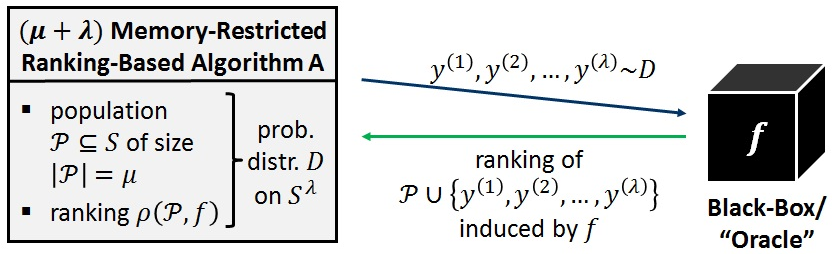A predominant topic in the theory of evolutionary algorithms and, more generally, theory of randomized black-box optimization techniques is running time analysis. Running time analysis aims at understanding the performance of a given heuristic on a given problem by bounding the number of function evaluations that are needed by the heuristic to identify a solution of a desired quality. As in general algorithms theory, this running time perspective is most useful when it is complemented by a meaningful complexity theory that studies the limits of algorithmic solutions. In the context of discrete black-box optimization, several black-box complexity models have been developed to analyze the best possible performance that a black-box optimization algorithm can achieve on a given problem. The models differ in the classes of algorithms to which these lower bounds apply. This way, black-box complexity contributes to a better understanding of how certain algorithmic choices (such as the amount of memory used by a heuristic, its selective pressure, or properties of the strategies that it uses to create new solution candidates) influences performance. In this chapter we review the different black-box complexity models that have been proposed in the literature, survey the bounds that have been obtained for these models, and discuss how the interplay of running time analysis and black-box complexity can inspire new algorithmic solutions to well-researched problems in evolutionary computation. We also discuss in this chapter several interesting open questions for future work.

* This survey article is to appear (in a slightly modified form) in the book "Theory of Randomized Search Heuristics in Discrete Search Spaces", which will be published by Springer in 2018. The book is edited by Benjamin Doerr and Frank Neumann. Missing numbers of pointers to other chapters of this book will be added as soon as possible
Click to Read Paper and Get Code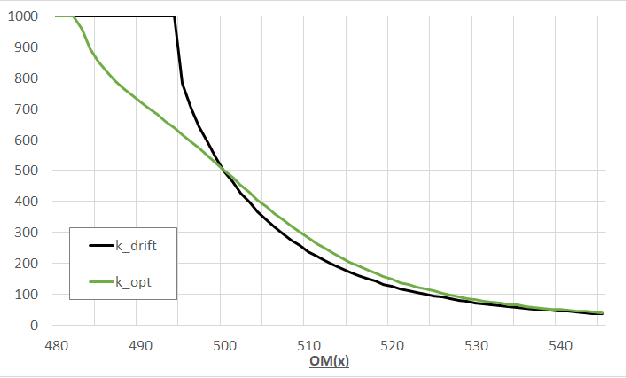It seems very intuitive that for the maximization of the OneMax problem $f(x):=\sum_{i=1}^n{x_i}$ the best that an elitist unary unbiased search algorithm can do is to store a best so far solution, and to modify it with the operator that yields the best possible expected progress in function value. This assumption has been implicitly used in several empirical works. In [Doerr, Doerr, Yang: GECCO 2016] it was formally proven that this approach is indeed almost optimal. In this work we prove that drift maximization is \emph{not} optimal. More precisely, we show that for most fitness levels $n/2<\ell/2 < 2n/3$ the optimal mutation strengths are larger than the drift-maximizing ones. This implies that the optimal RLS is more risk-affine than the variant maximizing the step-wise expected progress. We show similar results for the mutation rates of the classic (1+1) Evolutionary Algorithm (EA) and its resampling variant, the (1+1) EA$_{>0}$. As a result of independent interest we show that the optimal mutation strengths, unlike the drift-maximizing ones, can be even.

Click to Read Paper and Get Code
We show that for all $1<k \leq \log n$ the $k$-ary unbiased black-box complexity of the $n$-dimensional $\onemax$ function class is $O(n/k)$. This indicates that the power of higher arity operators is much stronger than what the previous $O(n/\log k)$ bound by Doerr et al. (Faster black-box algorithms through higher arity operators, Proc. of FOGA 2011, pp. 163--172, ACM, 2011) suggests. The key to this result is an encoding strategy, which might be of independent interest. We show that, using $k$-ary unbiased variation operators only, we may simulate an unrestricted memory of size $O(2^k)$ bits.

* An extended abstract of this paper has been accepted for inclusion in the proceedings of the Genetic and Evolutionary Computation Conference (GECCO 2012)
Click to Read Paper and Get Code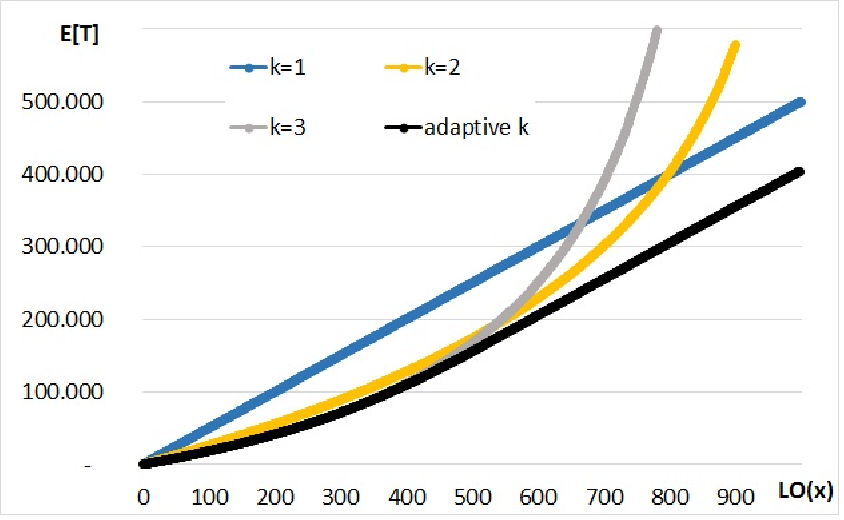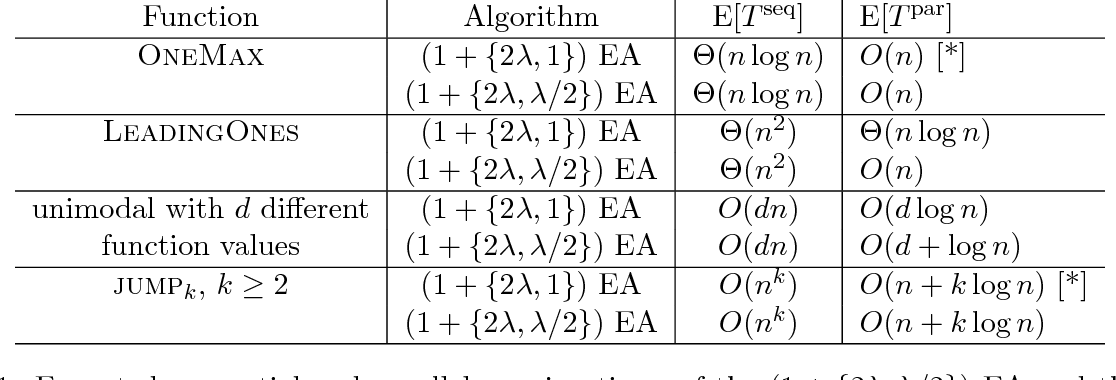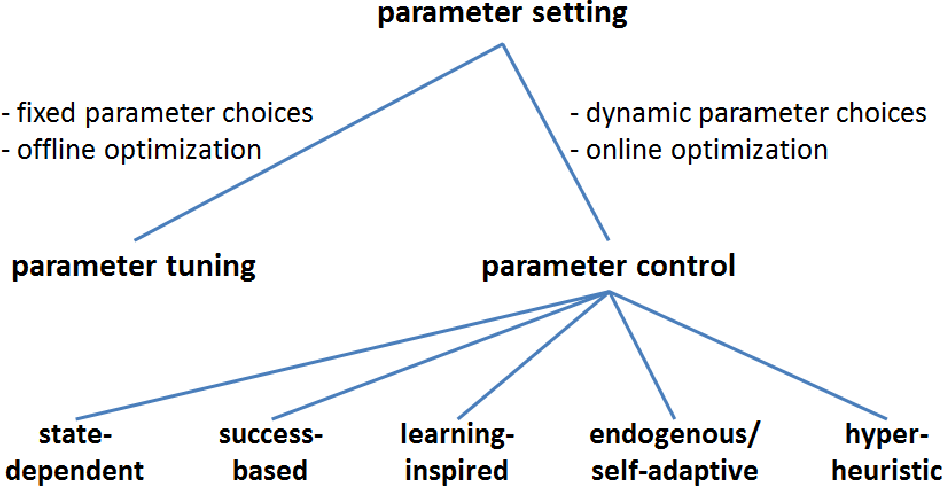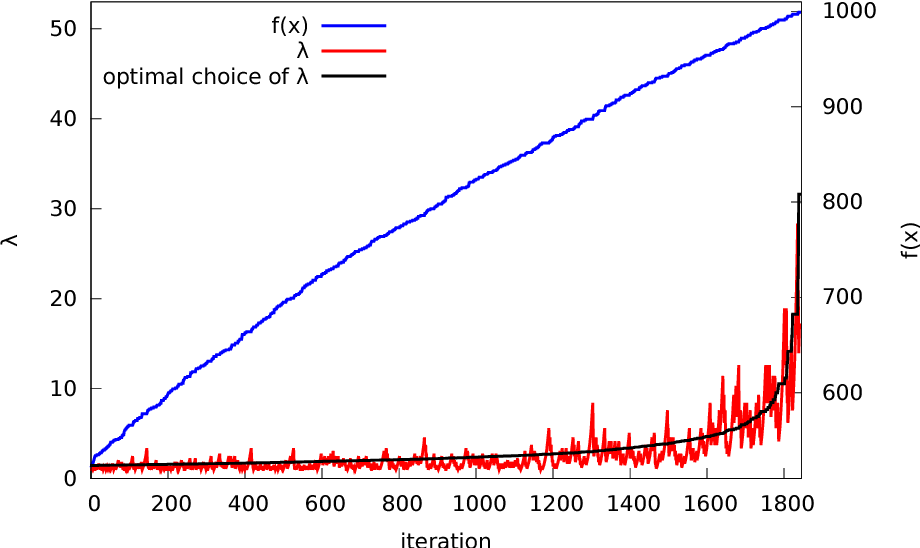Parameter control aims at realizing performance gains through a dynamic choice of the parameters which determine the behavior of the underlying optimization algorithm. In the context of evolutionary algorithms this research line has for a long time been dominated by empirical approaches. With the significant advances in running time analysis achieved in the last ten years, the parameter control question has become accessible to theoretical investigations. A number of running time results for a broad range of different parameter control mechanisms have been obtained in recent years. This book chapter surveys these works, and puts them into context, by proposing an updated classification scheme for parameter control.

* This book chapter is to appear in the book "Theory of Randomized Search Heuristics in Discrete Search Spaces", which is edited by Benjamin Doerr and Frank Neumann and is scheduled to be published by Springer in 2018
Click to Read Paper and Get Code
Motivated by a problem in the theory of randomized search heuristics, we give a very precise analysis for the coupon collector problem where the collector starts with a random set of coupons (chosen uniformly from all sets). We show that the expected number of rounds until we have a coupon of each type is $nH_{n/2} - 1/2 \pm o(1)$, where $H_{n/2}$ denotes the $(n/2)$th harmonic number when $n$ is even, and $H_{n/2}:= (1/2) H_{\lfloor n/2 \rfloor} + (1/2) H_{\lceil n/2 \rceil}$ when $n$ is odd. Consequently, the coupon collector with random initial stake is by half a round faster than the one starting with exactly $n/2$ coupons (apart from additive $o(1)$ terms). This result implies that classic simple heuristic called \emph{randomized local search} needs an expected number of $nH_{n/2} - 1/2 \pm o(1)$ iterations to find the optimum of any monotonic function defined on bit-strings of length $n$.

* Algorithmica 75 (2016), 529-553
Click to Read Paper and Get Code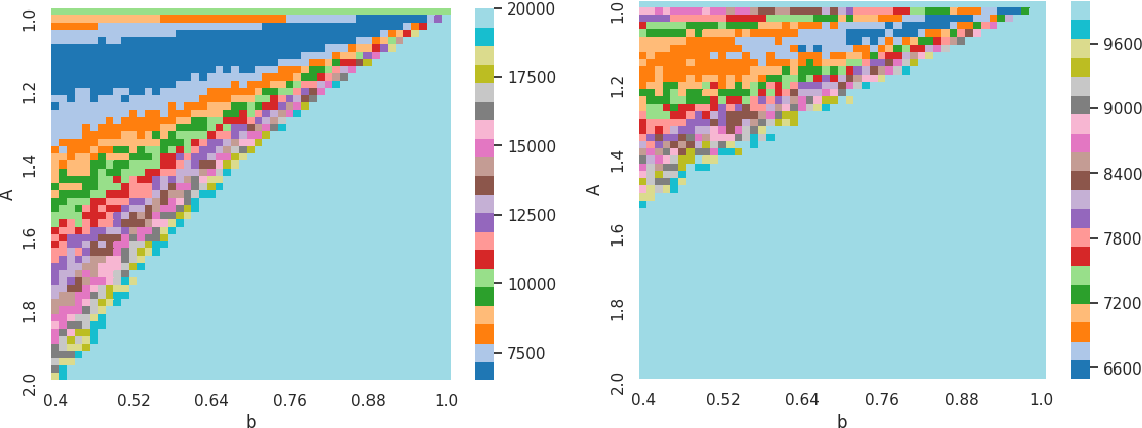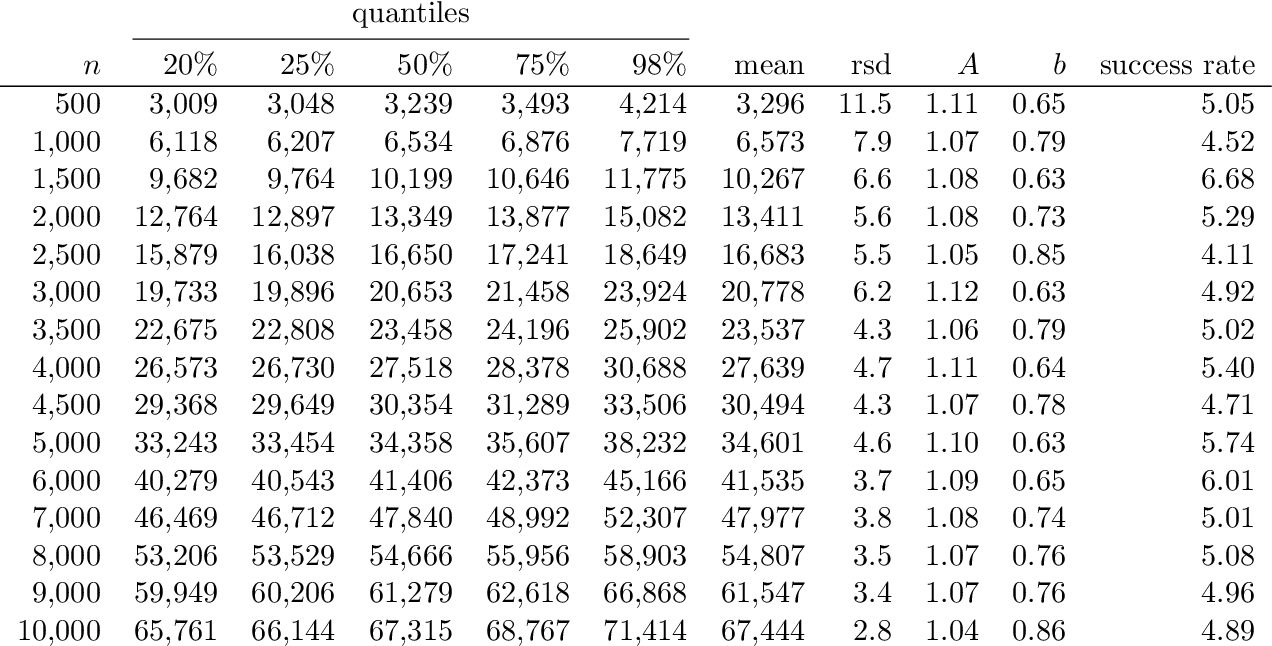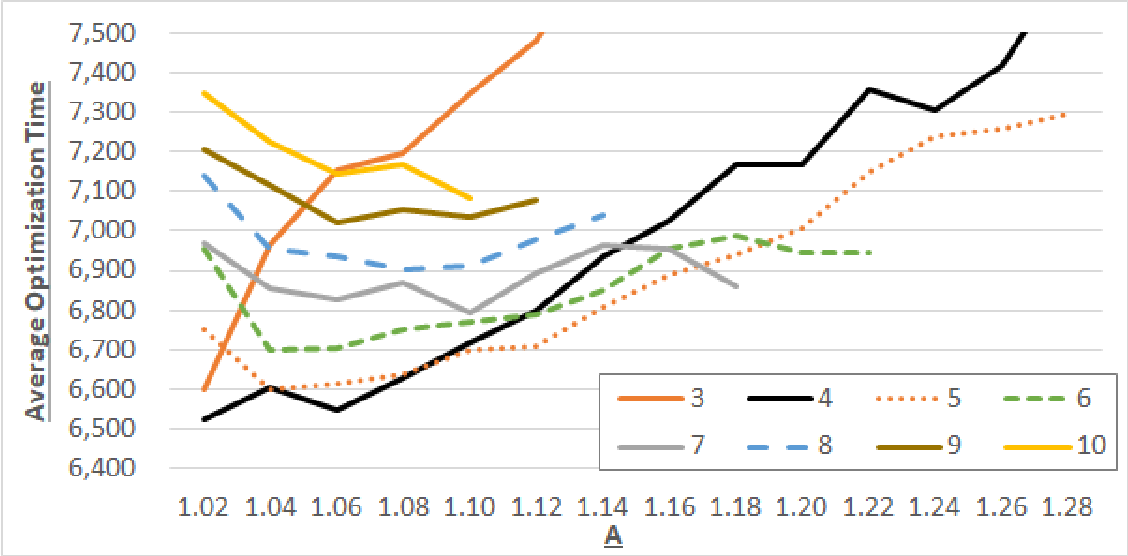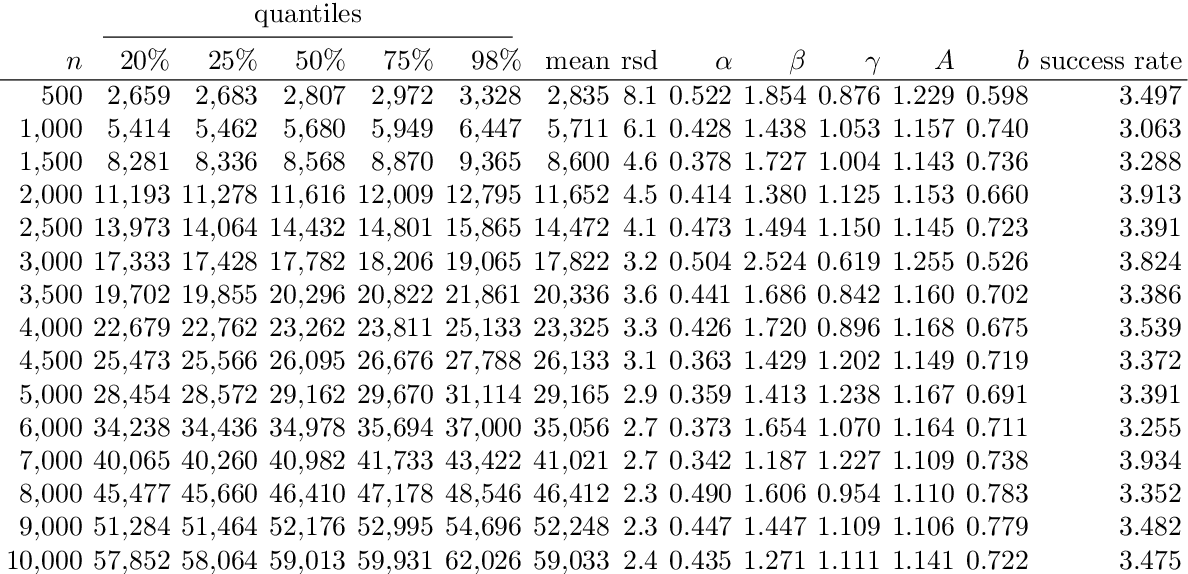It is known that the $(1+(\lambda,\lambda))$~Genetic Algorithm (GA) with self-adjusting parameter choices achieves a linear expected optimization time on OneMax if its hyper-parameters are suitably chosen. However, it is not very well understood how the hyper-parameter settings influences the overall performance of the $(1+(\lambda,\lambda))$~GA. Analyzing such multi-dimensional dependencies precisely is at the edge of what running time analysis can offer. To make a step forward on this question, we present an in-depth empirical study of the self-adjusting $(1+(\lambda,\lambda))$~GA and its hyper-parameters. We show, among many other results, that a 15\% reduction of the average running time is possible by a slightly different setup, which allows non-identical offspring population sizes of mutation and crossover phase, and more flexibility in the choice of mutation rate and crossover bias --a generalization which may be of independent interest. We also show indication that the parametrization of mutation rate and crossover bias derived by theoretical means for the static variant of the $(1+(\lambda,\lambda))$~GA extends to the non-static case.

* To appear at ACM Genetic and Evolutionary Computation Conference (GECCO'19). This version has some additional plots and data
Click to Read Paper and Get Code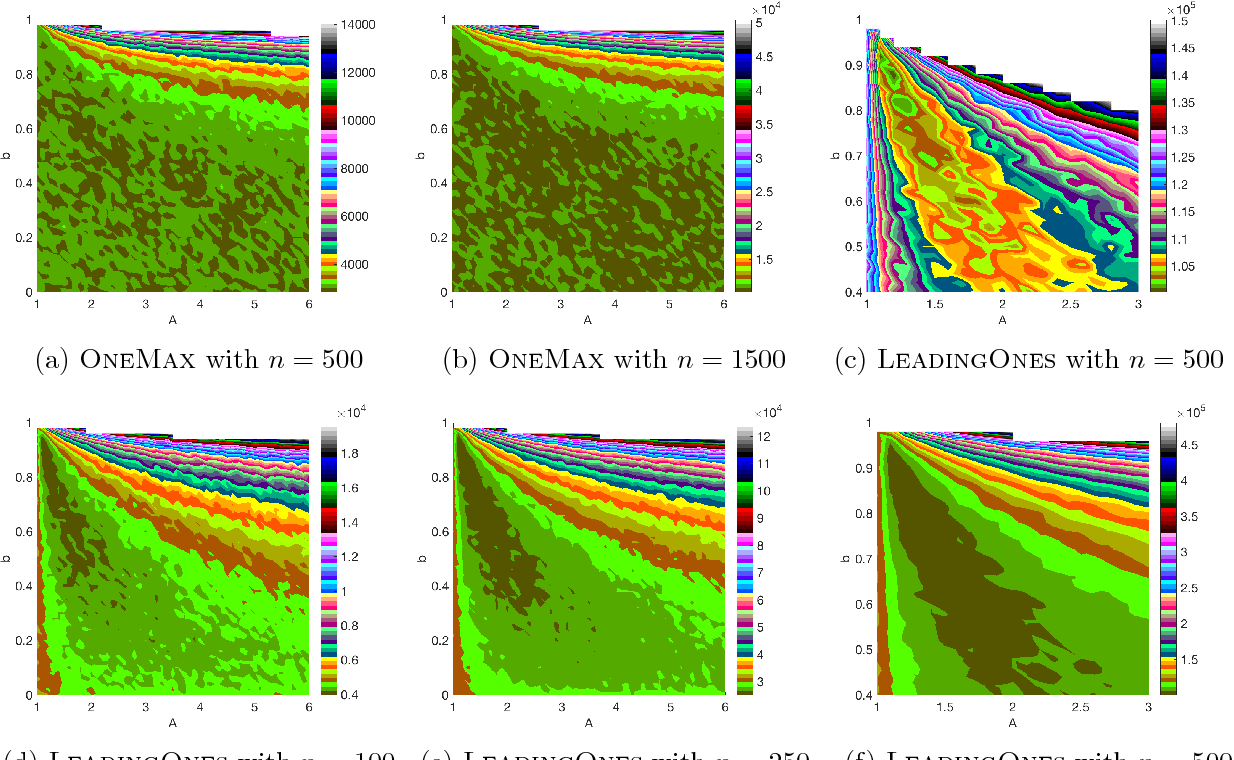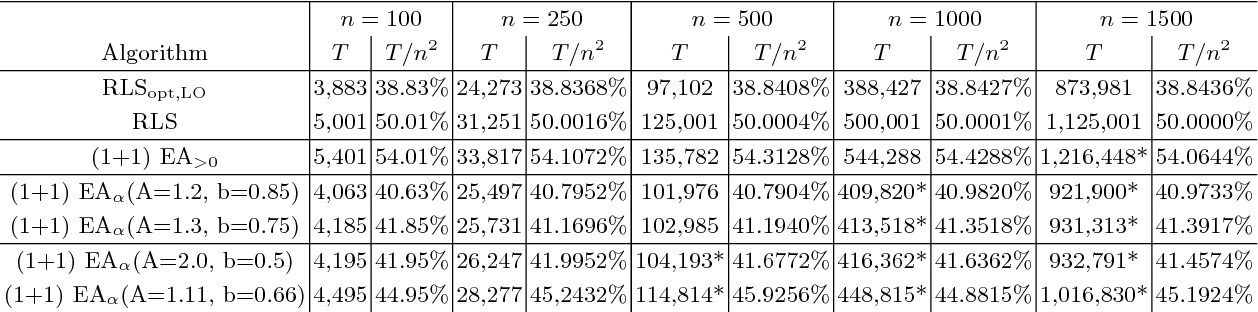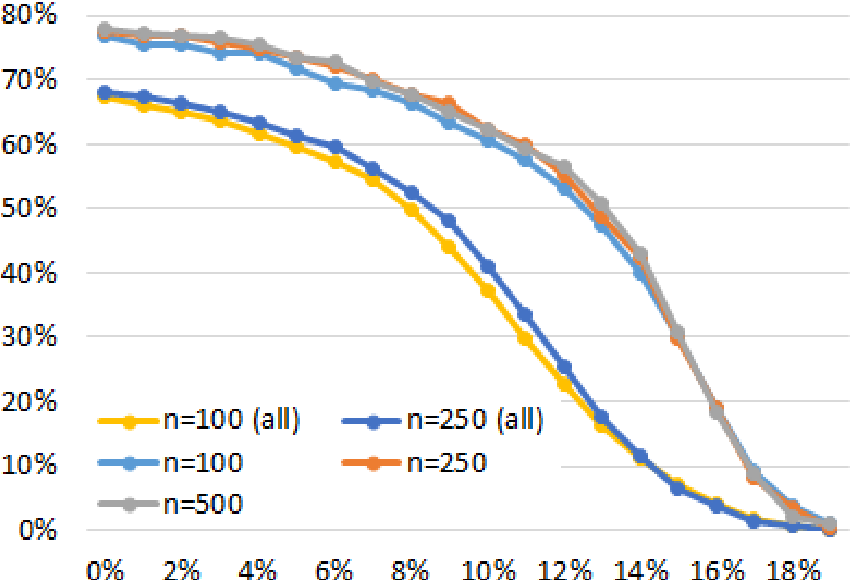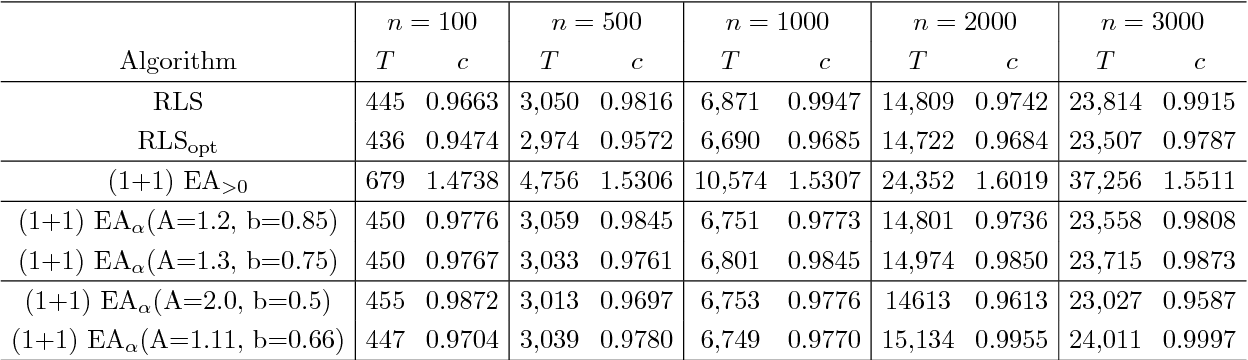Despite significant empirical and theoretically supported evidence that non-static parameter choices can be strongly beneficial in evolutionary computation, the question how to best adjust parameter values plays only a marginal role in contemporary research on discrete black-box optimization. This has led to the unsatisfactory situation in which feedback-free parameter selection rules such as the cooling schedule of Simulated Annealing are predominant in state-of-the-art heuristics, while, at the same time, we understand very well that such time-dependent selection rules can only perform worse than adjustment rules that do take into account the evolution of the optimization process. A number of adaptive and self-adaptive parameter control strategies have been proposed in the literature, but did not (yet) make their way to a broader public. A key obstacle seems to lie in their rather complex update rules. The purpose of our work is to demonstrate that high-performing online parameter selection rules do not have to be very complicated. More precisely, we experiment with a multiplicative, comparison-based update rule to adjust the mutation probability of a (1+1)~Evolutionary Algorithm. We show that this simple self-adjusting rule outperforms the best static unary unbiased black-box algorithm on LeadingOnes, achieving an almost optimal speedup of about~$18\%$.

Click to Read Paper and Get Code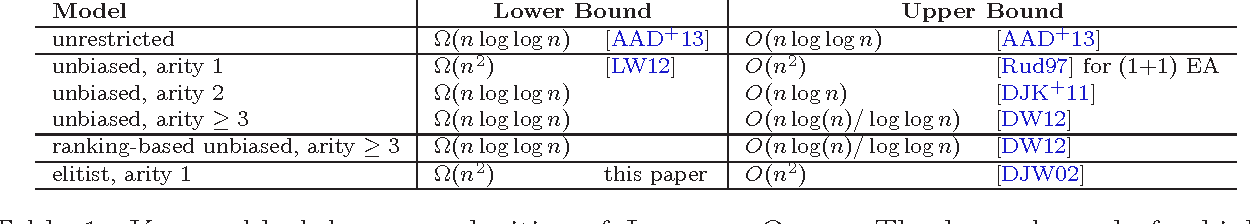One important goal of black-box complexity theory is the development of complexity models allowing to derive meaningful lower bounds for whole classes of randomized search heuristics. Complementing classical runtime analysis, black-box models help us understand how algorithmic choices such as the population size, the variation operators, or the selection rules influence the optimization time. One example for such a result is the $\Omega(n \log n)$ lower bound for unary unbiased algorithms on functions with a unique global optimum [Lehre/Witt, GECCO 2010], which tells us that higher arity operators or biased sampling strategies are needed when trying to beat this bound. In lack of analyzing techniques, almost no non-trivial bounds are known for other restricted models. Proving such bounds therefore remains to be one of the main challenges in black-box complexity theory. With this paper we contribute to our technical toolbox for lower bound computations by proposing a new type of information-theoretic argument. We regard the permutation- and bit-invariant version of \textsc{LeadingOnes} and prove that its (1+1) elitist black-box complexity is $\Omega(n^2)$, a bound that is matched by (1+1)-type evolutionary algorithms. The (1+1) elitist complexity of \textsc{LeadingOnes} is thus considerably larger than its unrestricted one, which is known to be of order $n\log\log n$ [Afshani et al., 2013].

* An extended abstract of this paper will appear at GECCO 2016
Click to Read Paper and Get Code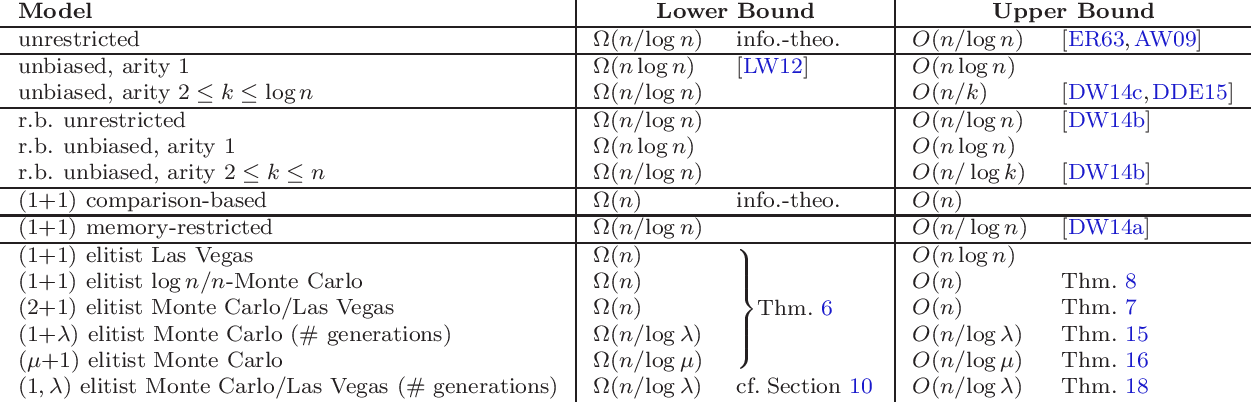Black-box complexity studies lower bounds for the efficiency of general-purpose black-box optimization algorithms such as evolutionary algorithms and other search heuristics. Different models exist, each one being designed to analyze a different aspect of typical heuristics such as the memory size or the variation operators in use. While most of the previous works focus on one particular such aspect, we consider in this work how the combination of several algorithmic restrictions influence the black-box complexity. Our testbed are so-called OneMax functions, a classical set of test functions that is intimately related to classic coin-weighing problems and to the board game Mastermind. We analyze in particular the combined memory-restricted ranking-based black-box complexity of OneMax for different memory sizes. While its isolated memory-restricted as well as its ranking-based black-box complexity for bit strings of length $n$ is only of order $n/\log n$, the combined model does not allow for algorithms being faster than linear in $n$, as can be seen by standard information-theoretic considerations. We show that this linear bound is indeed asymptotically tight. Similar results are obtained for other memory- and offspring-sizes. Our results also apply to the (Monte Carlo) complexity of OneMax in the recently introduced elitist model, in which only the best-so-far solution can be kept in the memory. Finally, we also provide improved lower bounds for the complexity of OneMax in the regarded models. Our result enlivens the quest for natural evolutionary algorithms optimizing OneMax in $o(n \log n)$ iterations.

* This is the full version of a paper accepted to GECCO 2015
Click to Read Paper and Get Code
Black-box complexity theory provides lower bounds for the runtime of black-box optimizers like evolutionary algorithms and serves as an inspiration for the design of new genetic algorithms. Several black-box models covering different classes of algorithms exist, each highlighting a different aspect of the algorithms under considerations. In this work we add to the existing black-box notions a new \emph{elitist black-box model}, in which algorithms are required to base all decisions solely on (a fixed number of) the best search points sampled so far. Our model combines features of the ranking-based and the memory-restricted black-box models with elitist selection. We provide several examples for which the elitist black-box complexity is exponentially larger than that the respective complexities in all previous black-box models, thus showing that the elitist black-box complexity can be much closer to the runtime of typical evolutionary algorithms. We also introduce the concept of $p$-Monte Carlo black-box complexity, which measures the time it takes to optimize a problem with failure probability at most $p$. Even for small~$p$, the $p$-Monte Carlo black-box complexity of a function class $\mathcal F$ can be smaller by an exponential factor than its typically regarded Las Vegas complexity (which measures the \emph{expected} time it takes to optimize $\mathcal F$).

* A short version of this work has been presented at the GECCO conference 2015 in Madrid, Spain. Available at http://dl.acm.org/citation.cfm?doid=2739480.2754654
Click to Read Paper and Get Code
We show that the unrestricted black-box complexity of the $n$-dimensional XOR- and permutation-invariant LeadingOnes function class is $O(n \log (n) / \log \log n)$. This shows that the recent natural looking $O(n\log n)$ bound is not tight. The black-box optimization algorithm leading to this bound can be implemented in a way that only 3-ary unbiased variation operators are used. Hence our bound is also valid for the unbiased black-box complexity recently introduced by Lehre and Witt (GECCO 2010). The bound also remains valid if we impose the additional restriction that the black-box algorithm does not have access to the objective values but only to their relative order (ranking-based black-box complexity).

* 12 pages, to appear in the Proc. of Artificial Evolution 2011, LNCS 7401, Springer, 2012. For the unrestricted black-box complexity of LeadingOnes there is now a tight $\Theta(n \log\log n)$ bound, cf. http://eccc.hpi-web.de/report/2012/087/
Click to Read Paper and Get Code
Randomized search heuristics such as evolutionary algorithms, simulated annealing, and ant colony optimization are a broadly used class of general-purpose algorithms. Analyzing them via classical methods of theoretical computer science is a growing field. While several strong runtime analysis results have appeared in the last 20 years, a powerful complexity theory for such algorithms is yet to be developed. We enrich the existing notions of black-box complexity by the additional restriction that not the actual objective values, but only the relative quality of the previously evaluated solutions may be taken into account by the black-box algorithm. Many randomized search heuristics belong to this class of algorithms. We show that the new ranking-based model gives more realistic complexity estimates for some problems. For example, the class of all binary-value functions has a black-box complexity of $O(\log n)$ in the previous black-box models, but has a ranking-based complexity of $\Theta(n)$. For the class of all OneMax functions, we present a ranking-based black-box algorithm that has a runtime of $\Theta(n / \log n)$, which shows that the OneMax problem does not become harder with the additional ranking-basedness restriction.

* This is an extended version of our CSR 2011 paper. 31 pages. The journal version is to appear in Algorithmica, DOI: 10.1007/s00453-012-9684-9
Click to Read Paper and Get Code
We analyze the classic board game of Mastermind with $n$ holes and a constant number of colors. A result of Chv\'atal (Combinatorica 3 (1983), 325-329) states that the codebreaker can find the secret code with $\Theta(n / \log n)$ questions. We show that this bound remains valid if the codebreaker may only store a constant number of guesses and answers. In addition to an intrinsic interest in this question, our result also disproves a conjecture of Droste, Jansen, and Wegener (Theory of Computing Systems 39 (2006), 525-544) on the memory-restricted black-box complexity of the OneMax function class.

* 23 pages
Click to Read Paper and Get Code
When a problem instance is perturbed by a small modification, one would hope to find a good solution for the new instance by building on a known good solution for the previous one. Via a rigorous mathematical analysis, we show that evolutionary algorithms, despite usually being robust problem solvers, can have unexpected difficulties to solve such re-optimization problems. When started with a random Hamming neighbor of the optimum, the (1+1) evolutionary algorithm takes $\Omega(n^2)$ time to optimize the LeadingOnes benchmark function, which is the same asymptotic optimization time when started in a randomly chosen solution. There is hence no significant advantage from re-optimizing a structurally good solution. We then propose a way to overcome such difficulties. As our mathematical analysis reveals, the reason for this undesired behavior is that during the optimization structurally good solutions can easily be replaced by structurally worse solutions of equal or better fitness. We propose a simple diversity mechanism that prevents this behavior, thereby reducing the re-optimization time for LeadingOnes to $O(\gamma\delta n)$, where $\gamma$ is the population size used by the diversity mechanism and $\delta \le \gamma$ the Hamming distance of the new optimum from the previous solution. We show similarly fast re-optimization times for the optimization of linear functions with changing constraints and for the minimum spanning tree problem.

* To appear at Genetic and Evolutionary Computation Conference (GECCO '19)
Click to Read Paper and Get Code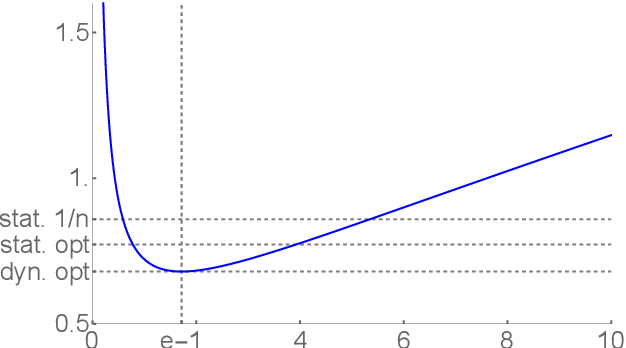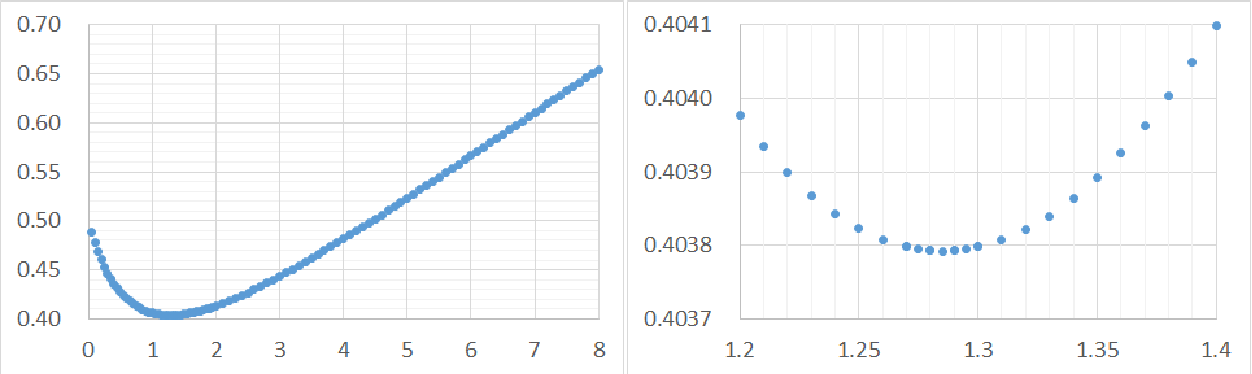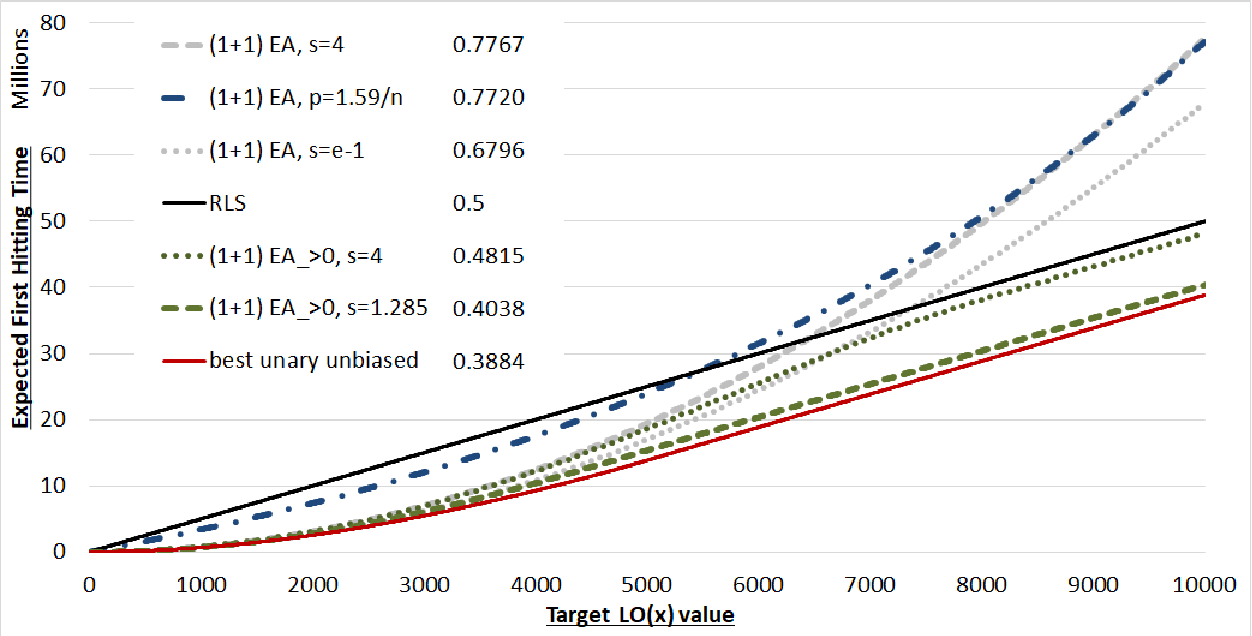The one-fifth success rule is one of the best-known and most widely accepted techniques to control the parameters of evolutionary algorithms. While it is often applied in the literal sense, a common interpretation sees the one-fifth success rule as a family of success-based updated rules that are determined by an update strength $F$ and a success rate $s$. We analyze in this work how the performance of the (1+1) Evolutionary Algorithm (EA) on LeadingOnes depends on these two hyper-parameters. Our main result shows that the best performance is obtained for small update strengths $F=1+o(1)$ and success rate $1/e$. We also prove that the running time obtained by this parameter setting is asymptotically optimal among all dynamic choices of the mutation rate for the (1+1) EA. We show similar results for the resampling variant of the (1+1) EA, which enforces to flip at least one bit per iteration.

Click to Read Paper and Get Code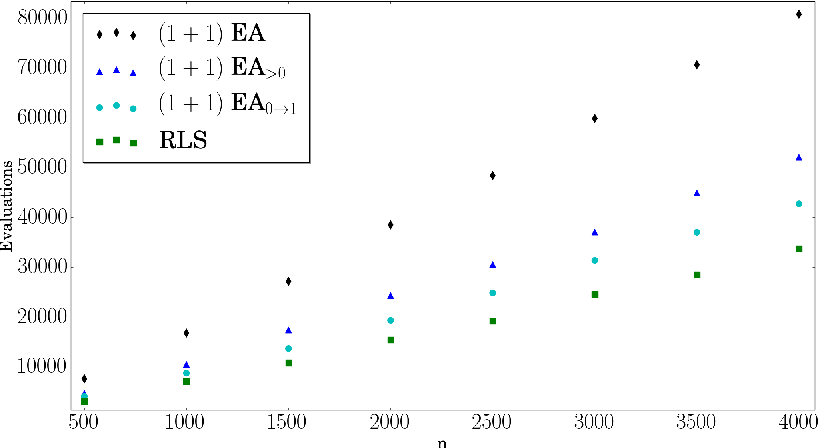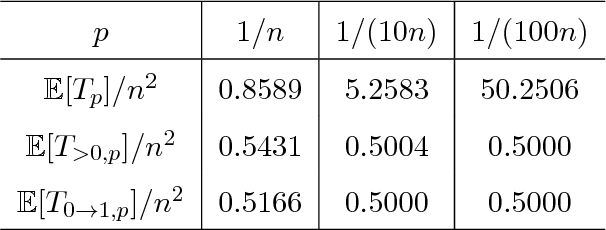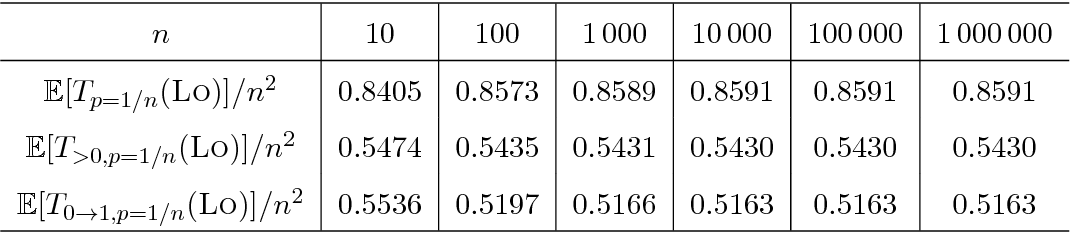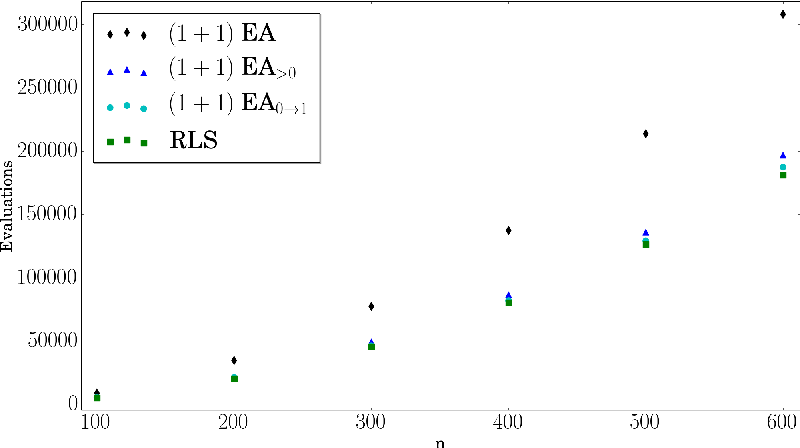Theory of evolutionary computation (EC) aims at providing mathematically founded statements about the performance of evolutionary algorithms (EAs). The predominant topic in this research domain is runtime analysis, which studies the time it takes a given EA to solve a given optimization problem. Runtime analysis has witnessed significant advances in the last couple of years, allowing us to compute precise runtime estimates for several EAs and several problems. Runtime analysis is, however (and unfortunately!), often judged by practitioners to be of little relevance for real applications of EAs. Several reasons for this claim exist. We address two of them in this present work: (1) EA implementations often differ from their vanilla pseudocode description, which, in turn, typically form the basis for runtime analysis. To close the resulting gap between empirically observed and theoretically derived performance estimates, we therefore suggest to take this discrepancy into account in the mathematical analysis and to adjust, for example, the cost assigned to the evaluation of search points that equal one of their direct parents (provided that this is easy to verify as is the case in almost all standard EAs). (2) Most runtime analysis results make statements about the expected time to reach an optimal solution (and possibly the distribution of this optimization time) only, thus explicitly or implicitly neglecting the importance of understanding how the function values evolve over time. We suggest to extend runtime statements to runtime profiles, covering the expected time needed to reach points of intermediate fitness values. As a direct consequence, we obtain a result showing that the greedy (2+1) GA of Sudholt [GECCO 2012] outperforms any unary unbiased black-box algorithm on OneMax.

* Internship report as of July 2017. Some references are outdated. Please get in touch if you are interested in a specific result and we will be happy to discuss the latest version
Click to Read Paper and Get Code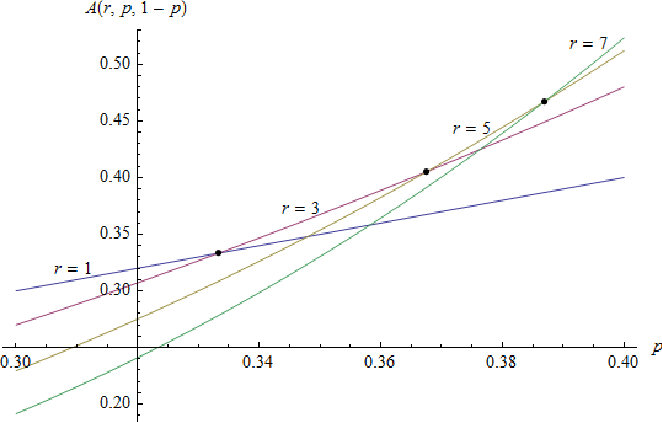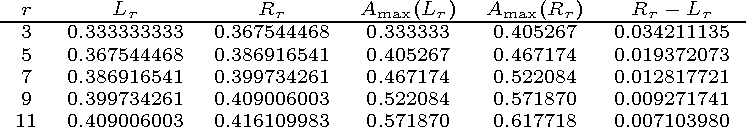It has been observed that some working principles of evolutionary algorithms, in particular, the influence of the parameters, cannot be understood from results on the asymptotic order of the runtime, but only from more precise results. In this work, we complement the emerging topic of precise runtime analysis with a first precise complexity theoretic result. Our vision is that the interplay between algorithm analysis and complexity theory becomes a fruitful tool also for analyses more precise than asymptotic orders of magnitude. As particular result, we prove that the unary unbiased black-box complexity of the OneMax benchmark function class is $n \ln(n) - cn \pm o(n)$ for a constant $c$ which is between $0.2539$ and $0.2665$. This runtime can be achieved with a simple (1+1)-type algorithm using a fitness-dependent mutation strength. When translated into the fixed-budget perspective, our algorithm finds solutions which are roughly 13\% closer to the optimum than those of the best previously known algorithms. To prove our results, we formulate several new versions of the variable drift theorems, which also might be of independent interest.

* Thoroughly revised version
Click to Read Paper and Get Code